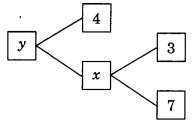# CBSEtips.in

## Thursday, 28 January 2021

### CBSE Class 10 Maths - MCQ Questions and Online Tests - Unit 1 - Triangles

#### CBSE Class 10 Maths – MCQ and Online Tests – Unit 1. Real Numbers

Every year CBSE conducts board exams for 10th standard. These exams are very competitive to all the students. So our website provides online tests for all the 10th subjects. These tests are also very effective and useful for those who preparing for competitive exams like NEET, JEE, CA etc. It can boost their preparation level and confidence level by attempting these chapter wise online tests.

These online tests are based on latest CBSE Class 10 syllabus. While attempting these our students can identify the weak lessons and continuously practice those lessons for attaining high marks. It also helps to revise the NCERT textbooks thoroughly.

#### CBSE Class 10 Maths – MCQ and Online Tests – Unit 1 – Real Numbers

Question 1.
For any positive integer a and b, there exist unique integers q and r such that a = 3q + r, where r must satisfy.
(a) 0 ≤ r < 3
(b) 1 < r < 3
(c) 0 < r < 3
(d) 0 < r ≤ 3

Answer: (a) 0 ≤ r < 3

Question 2.
The values of x and y is the given figure are(a) x + 10, y = 14
(b) x = 21, y = 84
(c) x = 21, y = 25
(d) x = 10, y = 40

Answer: (b) x = 21, y = 84

Question 3.
If HCF (a, b) = 12 and a × b = 1800 then LCM (a, b) is
(a) 3600
(b) 900
(c) 150
(d) 90

Question 4.
If mn = 32, where m and n are positive integers, then the value of (n)mn is
(a) 9765625
(b) 9775625
(c) 9785625
(d) 9865625

Question 5.
If ($$\frac{9}{7}$$)3 × ($$\frac{49}{81}$$)2x-6 = ($$\frac{7}{9}$$)9 then value of x is
(a) 12
(b) 9
(c) 8
(d) 6

Question 6.
The decimal expansion of $$\frac{17}{8}$$ will terminate after how many places of decimals?
(a) 1
(b) 2
(c) 3
(d) will not terminate

Question 7.
The decimal expansion of n is
(a) terminating
(b) non-terminating and non-recurring
(c) non-terminating and recurring
(d) does not exist.

Question 8.
If HCF of 55 and 99 is expressible in the form 55 m – 99, then the value of m:
(a) 4
(b) 2
(c) 1
(d) 3

Question 9.
Given that LCM of (91, 26) = 182 then HCF (91, 26) is
(a) 13
(b) 26
(c) 7
(d) 9

Question 10.
The decimal expansion of number $$\frac{441}{2^2×5^3×7}$$ is
(a) A terminating decimal
(b) Non-terminating but repeating
(c) Non-terminate non repeating
(d) terminating after two places of decimal

Question 11.
If A = 2n + 13, B = n + 7 where n is a natural number then HCF of A and B
(a) 2
(b) 1
(c) 3
(d) 4

Question 12.
(-1)n + (-1)8n = 0 when n is
(a) any positive integer
(b) any odd natural number
(c) any even numeral number
(d) any negative integer

Answer: (b) any odd natural number

Question 13.
If the LCM of 12 and 42 is 10 m + 4 then the value of m is
(a) 50
(b) 8
(c) $$\frac{1}{5}$$
(d) l

Question 14.
The decimal expansion of the rational number $$\frac{6243}{2^2×5^4}$$ will terminate after
(a) 4 places of decimal
(b) 3 places of decimal
(c) 2 places of decimal
(d) 1 place of decimal

Answer: (a) 4 places of decimal

Question 15.
n² – 1 is divisible by 8, if n is
(a) an integer
(b) a natural number
(c) an odd natural number
(d) an even natural number

Answer: (c) an odd natural number

Question 16.
If n is a natural number, then exactly one of numbers n, n + 2 and n + 1 must be a multiple of
(a) 2
(b) 3
(c) 5
(d) 7

Question 17.
The rational number between 72 and 73 is
(a) $$\frac{6}{5}$$
(b) $$\frac{3}{4}$$
(c) $$\frac{3}{2}$$
(d) $$\frac{4}{5}$$

Answer: (c) $$\frac{3}{2}$$

Question 18.
If a and 6 are two positive numbers and H and L are their HCF and LCM respectively. Then
(a) a × b = H × L
(b) a = b × H
(c) a = $$\frac{b×L}{H}$$
(d) H = $$\frac{L}{a×b}$$

Answer: (a) a × b = H × L

Question 19.
LCM of 2³ × 3² and 2² × 3³ is
(a) 2³
(b) 3³
(c) 2³ × 3³
(d) 2² × 3²

Question 20.
The LCM of 2.5, 0.5 and 0.175 is
(a) 2.5
(b) 5
(c) 7.5
(d) 0.875

Share: